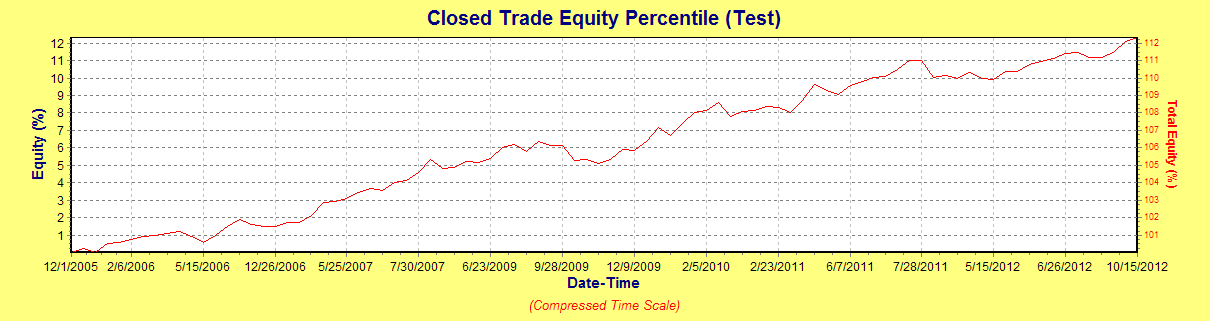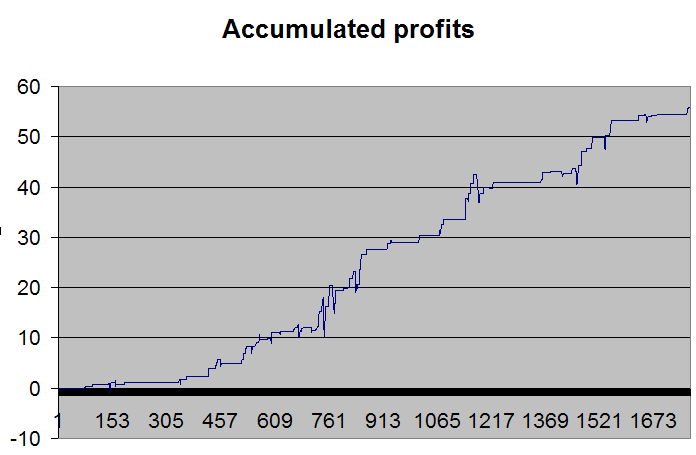# How To Make Money From The Close Until Tomorrow’s Open in SPY/S&P 500

Last Updated on June 11, 2021 by Oddmund Groette

This post has some simple suggestions on potential strategies from the close until tomorrow’s open in SPY (the test period is from October 2005 until the present).  This post is a summary of all several tests I have done on strategies with an entry on close and exit on the next day’s open (so far). The best strategy is at the end of this post and one I’ve been trading live for some months.

Here is the first strategy:

1. SPY closes at new 20 day low
2. Close is above the 200-day moving average.
3. (c-l)/(h-l)<0.5.
4. Go long at the close, exit at tomorrow’s open.

 P/L in % #trades #wins Avg. 8.4 35 29 0.24

The criteria about the close must be above the 200-day moving average is important. The equity curve gets a lot worse without it.

My favorite interval is 5 days (in SPY). Here is a new twist:

1. SPY closes at a new 5 day low.
2. Close is above the 200-day moving average.
3. (c-l)/(h-l) gives a ratio lower than 0.4.
4. Go long at the close, exit at tomorrow’s open.

 P/L in % #trades #wins Avg. 12.6 90 64 0.14

Most trades are below 0.4 (criteria 3) anyway. Here is the equity curve for this strategy:This is a pretty nice equity curve! The profit factor is 2.6.

What happens if we turn it upside down and go short? It gets a lot more erratic. There is a small negative edge, but not tradeable. However, nice to know, though (not to go long these times).

I also tested this using  RSI(5):

1. RSI(5) is below 25.
2. Close is above the 200-day moving average.
3. (c-l)/(h-l) gives a ratio lower than 0.5.
4. Go long at the close, exit at tomorrow’s open.

 P/L in % #trades #wins Avg. 13.57 59 46 0.23

The equity curve is just as upward sloping as the one above and has a profit factor of about 3.8. Here is the annual return trading 490 000 USD per position:Here is another twist which I believe is the best, considering it has more fills:

1. (c-l)/(h-l)<0.5
2. RSI(5)<30
3. Close is lower than the 50-day moving average

 P/L in % #trades #wins Avg. 36.55 168 109 0.23

This is quite impressive. Why? Probably because the market is short-term oversold and get a quick bounce up from a bad day. Buying if the close is above the 50-day average is no good at all using these criteria. Quite a difference from the above strategies that went long if above 200 the day moving average. If holding to the close instead of selling at the open, the total P/L increases to 80% but the equity curve is a lot more erratic. So buying on a 5 day low is a lot better for holding several days.

From previous research, I know there is a good chance of filling the gap if SPY opens down and is short-term oversold. So I put in a new twist: if SPY opens lower (the next day) than -0.1%, simply hold until the close (but exit at the open if opens higher than -0.1%). This boosts the strategy:

 P/L in % #trades #wins Avg. 55.81 168 129 0.33

Here is the profit curve of this strategy:In the last years, it has paid off to hold this position until the next day’s close even on days when SPY opens up. But this was not the case earlier.

I think the last strategy is pretty good! Combined with others it can boost performance. Sometimes there is overlap with the 5 day low strategy, but not that often.